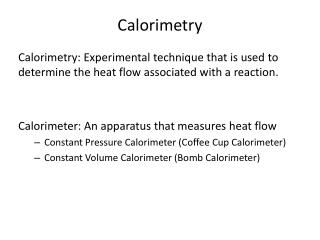DownloadDownload PresentationCalorimetry

Calorimetry

Download PresentationCalorimetry

- - - - - - - - - - - - - - - - - - - - - - - - - - - E N D - - - - - - - - - - - - - - - - - - - - - - - - - - -
Presentation Transcript

1. Calorimetry Calorimetry: Experimental technique that is used to determine the heat flow associated with a reaction. Calorimeter: An apparatus that measures heat flow • Constant Pressure Calorimeter (Coffee Cup Calorimeter) • Constant Volume Calorimeter (Bomb Calorimeter)

2. Measuring DHCalorimetry at Constant Pressure • Reaction is carried out in an aqueous solution in a simple calorimeter • Measure the heat change for the system by measuring the temperature change for water in the calorimeter. • Because the specific heat for water is well known (4.184 J/g-K), we can measure H for the reaction with this equation. q = ΔHreaction = (masssolution x Cs, solution x ΔT) • To calculate ΔHreaction per mol, divide ΔHreaction by the number of moles Figure 5.18 Coffee-cup calorimeter

3. Practice: Calcualting ΔH using a Coffee-Cup Calorimeter When a student mixes 50.0 mL of 1.0 M HCl and 50.0 mL of 1.0 M NaOH in a coffee cup calorimeter, the temperature of the resultant solution increases from 21.0 °C to 27.5 °C. Calculate the ΔHrxnin kJ/mol HCl, assuming that the calorimeter loses only a negligible amount of heat, that the total volume of the solution is 100 mL with density 1.0 g/mL. The specific heat of the solution is 4.18 J/g °C . (Ans: -54 kJ/molHCl)

4. Measuring Specific Heat of an Unknown metal Using Coffee Cup Calorimeter • Heat the unknown metal in boiling water for approximately 10 minutes and transfer this hot metal to the coffee cup calorimeter. • When the hot piece of metal is placed into the coffee cup calorimeter containing room temperature water, a spontaneous heat transfer occurs from the hot metal to the water and the coffee cup calorimeter • Measure the temperature change of water • Heat lost by hot metal = heat gained by cold water + heat gained by the calorimeter qmetal = qwater + q calorimeter • Use the above equation to calculate specific heat of metal

5. Practice: Calculation of Specific Heat using Coffee Cup Calorimeter A 110. g sample of copper was heated to 82.4 °C and placed into 116.0 g of water at 22.3 °C. The temperature of water increased to 24.9 °C. What is the specific heat of copper? Assume heat gained by the calorimeter is zero. (sp ht capacity of water = 4.18 J/g °C)(Ans: 0.20 J/g °C)